# An Introduction to SEMinR Package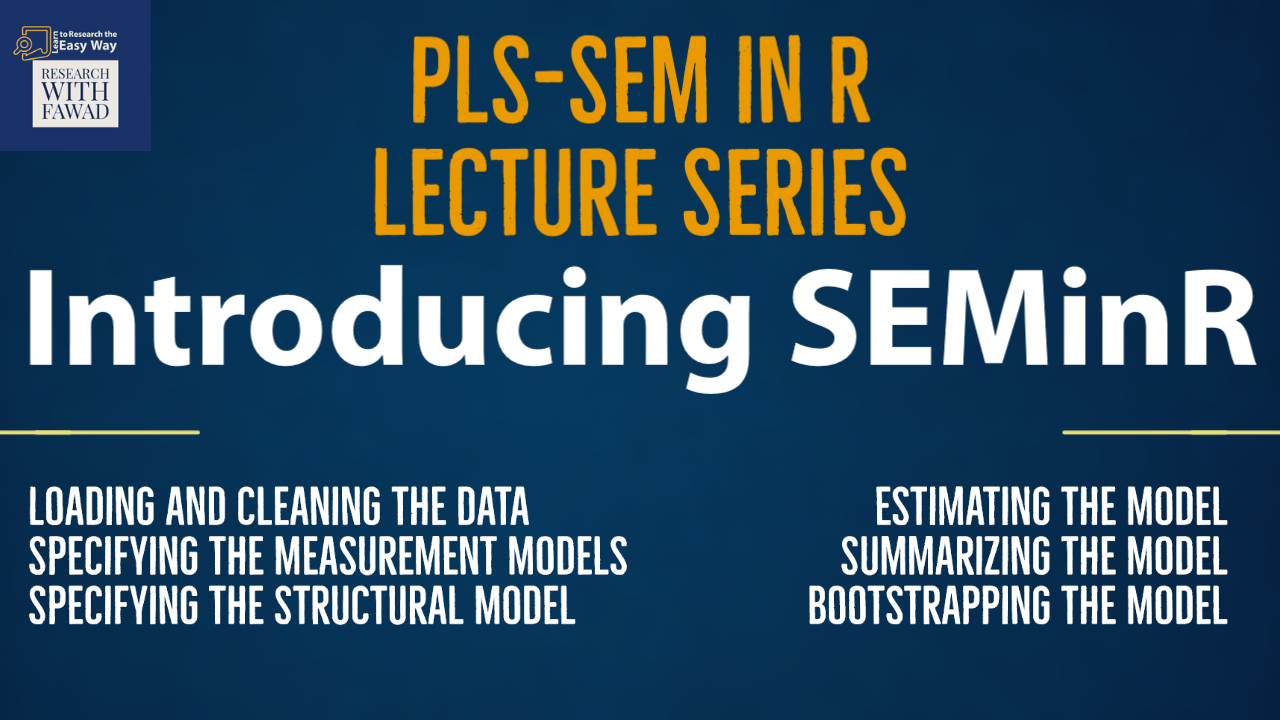### Introduction to SEMinR

This session on on SEMinR Package will focus on

• Specifying the Measurement Models
• Specifying the Structural Model
• Estimating the Model
• Summarizing the Model
• Bootstrapping the Model

## Understanding SEMinR Package

• SEMinR is a software package developed for the R statistical environment (R Core Team, 2021) that brings a user-friendly syntax to creating and estimating structural equation models.
• SEMinR is open source, which means that anyone can inspect, modify, and enhance the source code.
• Users of SEMinR can also interact with the developers and each other at the Facebook group (https:// www.facebook.com/ groups/seminr).
• The SEMinR syntax enables applied practitioners of PLS-SEM to use terminology that is very close to their familiar modeling terms (e.g., reflective, composite, and interactions), instead of specifying underlying matrices and covariances.
``````# Download and install the SEMinR package
# You only need to do this once to equip
# Rstudio on your computer with SEMinR
install.packages(“seminr”)
# Make the SEMinR library ready to use
# You must do this every time you restart Rstudio and wish to use SEMinR
library(seminr)``````

## SEMinR

There are four steps to specify and estimate a structural equation model using SEMinR:

• Specifying the measurement models
• Specifying the structural model
• Estimating, bootstrapping, and summarizing the model

• When estimating a PLS-SEM model, SEMinR expects you to have already loaded your data into an object. This data object is usually a data.frame class object.
• The read.csv() function allows you to load data into R if the data file is in a .csv (comma-separated value) or .txt (text) format. Note that there are other packages that can be used to load data in Microsoft Excel’s .xlsx format or other popular data formats.
• Comma-separated value (CSV) files are a type of text file, whose lines contain the data of each subject or case of your dataset.
• The values are typically separated by commas but can also be separated by other special characters (e.g., semicolons).
• The first line of the file typically consists of variable names, called the header line, and is also separated by commas or other special characters.
• Thus, a variable will have its name in the first row and its values will be in all the following lines of data at the same position.
• Many software packages, such as Microsoft Excel and SPSS, can export data into a .csv format.
• We can load data from a .csv file using the read.csv().
• Remember that you can use the ? operator to find help about a function in R (e.g., use ?read. csv).
• Table shows several arguments for the read.csv().
• In this section, we will demonstrate how to load a .csv file into the Rstudio global environment.
• The comma (,) is used as a separator character, and the missing values are coded as −99.
• If you wish to import this file to the global environment, you can use the read.csv() function,
``````#Step 1
library(seminr)
``````

When Data file is not in the same folder as R Script

``````#Data File not in the Sample Folder
``````

Important

• Inspect the loaded data to ensure that the correct numbers of columns (indicators), rows (observations or cases), and column headers (indicator names) appear in the loaded data.
• Note that SEMinR uses the asterisk (“*”) character when naming interaction terms as used in, for example, moderation analysis, so please ensure that asterisks are not present in the indicator names.
• Duplicate indicator names will also cause errors in SEMinR. Finally, missing values should be represented with a missing value indicator (such as −99, which is commonly used), so they can be appropriately identified and treated as missing values.
• We will use head() function to inspect the data.
• It is clear from inspecting the head of the data object () that the file has been loaded correctly and has the value “-99” set for the missing values.
• With the data loaded correctly, we now turn to the measurement model specification.
``````#To Inspect Data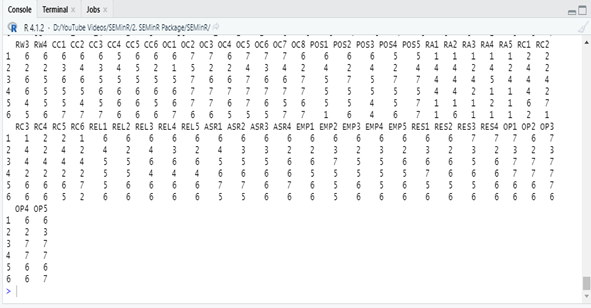## Step 2: Specify the Measurement Model

• Path models are made up of two elements:
• The measurement models (also called outer models in PLS-SEM), which describe the relationships between the latent variables and their measures (i.e., their indicators), and
• The structural model (also called the inner model in PLS-SEM), which describes the relationships between the latent variables. We begin with describing how to specify the measurement models.
• Measurement model is assessed to establish the quality criteria (Reliability and Validity).
• Hypothesis tests involving the structural relationships among constructs will only be as reliable or valid as the construct measures.
• SEMinR uses the constructs() function to specify the list of all construct measurement models. Within this list, various constructs can be defined using:
• composite() specifies the measurement of individual constructs.
• interaction_term() specifies interaction terms.
• higher_composite() specifies hierarchical component models (higher-order constructs; Sarstedt et al., 2019).
• The constructs() function compiles the list of constructs and their respective measurement model definitions.
• We must supply it with any number of individual composite(), interaction_term(), or higher_composite() constructs using their respective functions.
• The composite() function describes the measurement model of a single construct and takes the arguments shown in Table.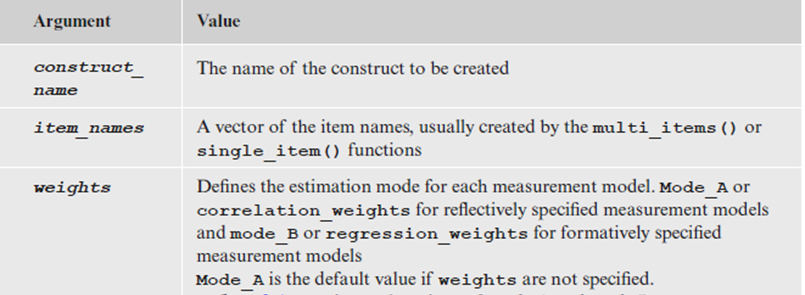• SEMinR strives to make specification of measurement items shorter and cleaner using multi_items(), which creates a vector of multiple measurement items with similar names or single_item() that describes a single measurement item.
• A vector is a sequence of data elements of the same basic type. Members in a vector are officially called components. Vectors in R are the same as the arrays in C language which are used to hold multiple data values of the same type.
• For example, we can use composite() for PLS path models to describe the reflectively measured Constructs

composite(“Put in Construct Name in Quotes”, multi_items(“Construct Code”, Starting Number:Ending Number), weights = mode_A);

Collaborative Culture construct with its indicator variables CC1, CC2, CC3, CC4, CC5, CC6:

• Explanations of mode A and mode B are discussed later. When no measurement weighting scheme is specified, the argument default is set to mode_A.
``composite(“Collaborative Culture”, multi_items(“CC”, 1:6), weights = mode_A);``
• Similarly, if you have a single item construct, you can use composite() to define the single-item measurement model as

composite(“CUSA”, single_item(“cusa”))

• Using composite define your constructs in the mode, next, combine the measurement models within the constructs() function, we can define the measurement model for the simple model like using constructs and composite (see next slide).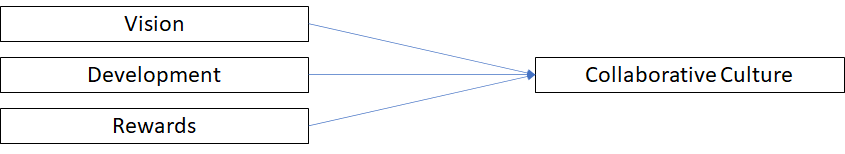Note: If an error occurs, make sure you used the library(seminr) command in R to load the SEMinR package before executing the program code.

The program code facilitates the specification of standard measurement models. However, the constructs() function also allows specifying more complex models, such as interaction terms (Memon et al., 2019) and higher-order constructs (Sarstedt et al., 2019). We will discuss the interaction_term() function for specifying interactions in more detail later.

Step 2 in Creating a Model – Identify the variables in your study and Put them as Measurement Model.

``````#Step 2: Create measurement model
simple_mm <- constructs(
composite("Vision", multi_items("VIS", 1:4)),
composite("Development", multi_items("DEV", 1:7)),
composite("Rewards", multi_items("RW",1:4)),
composite("Collaborative Culture", multi_items("CC", 1:6)))``````

Here simple_mm is an object which stores the constructs in the study.

<- Can be considered as an equal sign that assigns the constructs to the object.

constructs function holds the variables from the study, defined as composite (as discussed in the last slide)

## Step 3: Specifying the Structural Model

• With our measurement model specified, we now specify the structural model. When a structural model is being developed, two primary issues need to be considered: the sequence of the constructs and the relationships between them.
• Both issues are critical to the concept of modeling because they represent the hypotheses and their relationships to the theory being tested.
• In most cases, researchers examine linear independent–dependent relationships between two or more constructs in the path model.
• SEMinR makes structural model specification more human readable, domain relevant, and explicit by using these functions:
• relationships() specifies all the structural relationships between all constructs.
• paths() specifies relationships between sets of antecedents and outcomes.
• The simple model shown earlier has three relationships. For example, to specify the relationships from Vision, Development, and Rewards to Collaborative Culture, we use the from and to arguments in the path function:

paths(from = c(“Vision”, “Development”, “Rewards”), to = “Collaborative Culture”).

``````#Step 3: Create structural model
simple_sm <- relationships(
paths(from = c("Vision", "Development", "Rewards"), to = "Collaborative 	Culture"))
c can be used when specifying both multiple or single construct in a relationship
``````

Here simple_sm is an object which stores the relationships in the study.

<- Can be considered as an equal sign that assigns the constructs to the object.

relationships function holds the proposed relationships identified as individual paths

The code mentioned above, is the depiction of the following framework.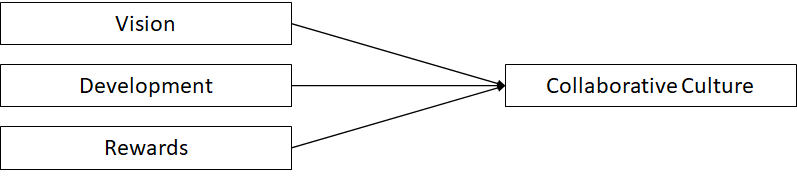## Step 4: Estimating the Model

Step 3 in creating a model

• After having specified the measurement and structural models, the next step is the model estimation using the PLS-SEM algorithm.
• For this task i-e estimation, the algorithm helps in determing the scores of the constructs that are later used as input for (single and multiple) regression models within the path model.
• After the algorithm has calculated the construct scores, the scores are used to estimate each regression model in the path model.
• As a result, we obtain the estimates for all relationships in the measurement models (i.e., the indicator weights/loadings) and the structural model (i.e., the path coefficients).
• To estimate a PLS path model, algorithmic options and argument settings must be selected. The algorithmic options and argument settings include selecting the structural model path weighting scheme. SEMinR allows the user to apply two structural model weighting schemes:
• The factor weighting scheme and
• The path weighting scheme.
• While the results differ little across the alternative weighting schemes, path weighting is the most popular and recommended approach.
• This weighting scheme provides the highest R-Sq value for endogenous latent variables and is generally applicable for all kinds of PLS path model specifications and estimations.
• SEMinR uses the estimate_pls() function to estimate the PLS-SEM model.
• This function applies the arguments shown in . Table. Please note that arguments with default values do not need to be specified but will revert to the default value when not specified.

•

We now estimate the PLS-SEM model by using the estimate_pls() function with arguments

data = datas,

measurement_model = simple_mm,

structural_model = simple_sm,

inner_weights = path_weighting,

missing = mean_replacement, and

missing_value = “-99”

and assign the output to simple_model.

• It is like running PLS Algorithm in SmartPLS

``````# Estimate the model
simple_model <- estimate_pls(data = datas,
measurement_model = simple_mm,
structural_model = simple_sm,
inner_weights = path_weighting,
missing= mean_replacement,
missing_value = "-99")``````

Note that the arguments for inner_weights, missing, and missing_value can be omitted if the default arguments are used. This is equivalent to the previous code block:

``````# Estimate the model with Omissions
simple_model <- estimate_pls(data = datas,
measurement_model = simple_mm,
structural_model = simple_sm)``````

## Bootstrapping

• PLS-SEM is a nonparametric method – thus, we need to perform bootstrapping to estimate standard errors and compute confidence intervals.
• The bootstrap_model() function is used to bootstrap a previously estimated SEMinR model (simple_model). The previously estimated pls model (the object holding the pls estimation is bootstrapped)
• This function applies the arguments shown in Table. In the example, we use the bootstrap_model() function and specify the arguments seminr_model = simple_model, nboot = 1000, cores = NULL, seed = 123.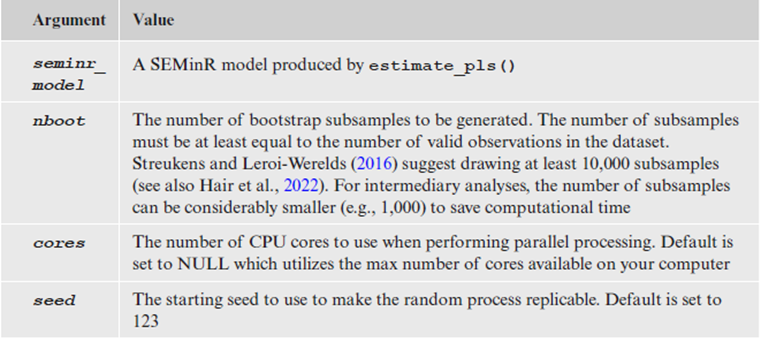• In this example, we use 1,000 bootstrap subsamples. However, the final result computations should draw on 10,000 subsamples (Streukens & Leroi-Werelds, 2016).
• We first assign the output of the bootstrapping to the boot_simple variable.
``````# Bootstrap the model
boot_simple <- bootstrap_model
(seminr_model = simple_model,
nboot = 1000,
cores = NULL,
seed = 123)``````
• We then summarize this variable, assigning the output of summary() to the summary_boot variable.
• The summarized bootstrap model object (i.e., summary_boot) contains the elements shown in . Table, which can be inspected using the \$ operator.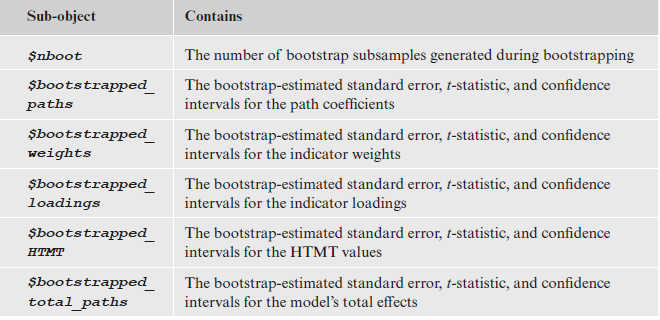``````# Store the summary of the bootstrapped model
summary_boot <- summary(boot_simple)
#Retreive Full Report
summary_boot
# Inspect the bootstrapped structural paths
summary_boot\$bootstrapped_paths

## Review of the Steps

Following is a brief review of the steps that have been discussed in SEMinR tutorials.

• Load the Library – library ()
• Review the Data – head()
• Specify the Measurement Model – constructs()
• Specify the Structural Model – relationships()
• Estimate the Model – estimate_pls()
• Summarize the Results – summary()
• Bootstrap the Model – bootstrap_model()
• Summarize the Results – summary()

The next step is Plotting and Writing Results – plot() and Write.csv

## Complete Code

``````#Loading the Library
library(seminr)
#To Inspect Data
#Create measurement model
simple_mm <- constructs(
composite("Vision", multi_items("VIS", 1:4)),
composite("Development", multi_items("DEV", 1:7)),
composite("Rewards", multi_items("RW",1:4)),
composite("Collaborative Culture", multi_items("CC", 1:6)))
# Create structural model
simple_sm <- relationships(
paths(from = c("Vision", "Development", "Rewards"), to = "Collaborative Culture"))
# Estimate the model
simple_model <- estimate_pls(data = datas,
measurement_model = simple_mm, structural_model = simple_sm,
inner_weights = path_weighting,
missing = mean_replacement,
missing_value = "-99")
# Summarize the model results
summary_simple <- summary(simple_model)
#Inspect the Summary Report
summary_simple
# Inspect the model’s path coefficients and the R^2 values
summary_simple\$paths
# Inspect the construct reliability metrics
summary_simple\$reliability
# Bootstrap the model
boot_simple <- bootstrap_model(seminr_model = simple_model,
nboot = 1000,
cores = NULL,
seed = 123)
# Store the summary of the bootstrapped model
summary_boot <- summary(boot_simple)
#Retreive Full Report
summary_boot
# Inspect the bootstrapped structural paths
summary_boot\$bootstrapped_paths